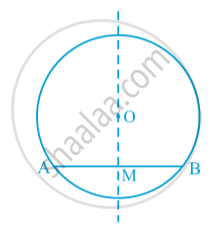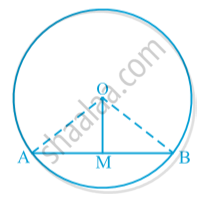# Perpendicular from the Centre to a Chord

#### theorem

Theorem : The perpendicular from the centre of a circle to a chord bisects the chord.Draw a circle. Let O be its centre. Draw a chord AB. Let the crease cut AB at the point M. Then, ∠ OMA = ∠ OMB = 90° or OM is perpendicular to AB.  So MA = MB.

Theorem :  The line drawn through the centre of a circle to bisect a chord is perpendicular to the chord.
Let AB be a chord of a circle with centre O and O is joined to the mid -point M of AB.
To prove: OM⊥ AB.Proof: Join OA and OB
In triangles OAM and OBM,
OA =OB (radii of the same circle)
AM =BM (M is the midpoint of PQ)
OM =OM (common)
Therefore, ∆OAM ≅ ∆OBM   (SSS congruence rule)
This gives , ∠OMA = ∠OMB = 90°
(Corresponding parts of congruent triangles are congruent).

If you would like to contribute notes or other learning material, please submit them using the button below.

### Shaalaa.com

Theorem : The perpendicular from the centre of a circle to a chord bisects the chord. [00:12:09]
S
0%